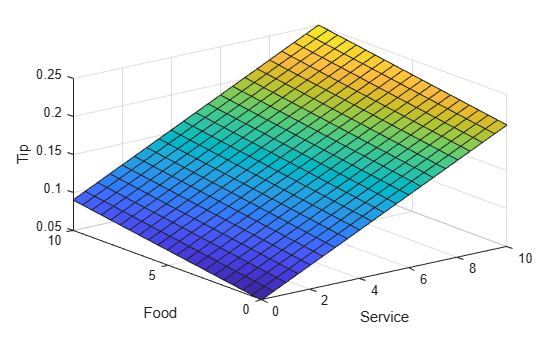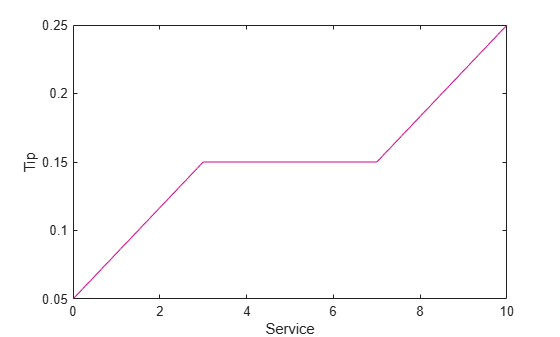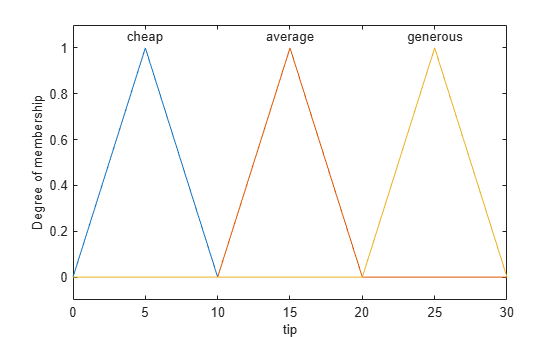# Fuzzy vs. Nonfuzzy Logic

### Basic Tipping Problem

To illustrate the value of fuzzy logic, examine both linear and fuzzy approaches to the following problem:

What is the right amount to tip your waitperson?

First, work through this problem the conventional (nonfuzzy) way, writing MATLAB® commands that spell out linear and piecewise-linear relations. Then, look at the same system using fuzzy logic.

Basic Tipping Problem. Given a number from 0 through 10 that represents the quality of service at a restaurant (where 10 is excellent), what should the tip be?

This problem is based on tipping as it is typically practiced in the United States. An average tip for a meal in the US is 15%, though the actual amount can vary depending on the quality of the service provided.

### Nonfuzzy Approach

Begin with the simplest possible relationship. Suppose that the tip always equals 15% of the total bill.

```service = 0:.5:10; tip = 0.15*ones(size(service)); plot(service,tip) xlabel('Service') ylabel('Tip') ylim([0.05 0.25])```This relationship does not account for the quality of the service, so you must add a term to the equation. Since service is rated on a scale from 0 through 10, you the tip increase linearly from 5% if the service is bad to 25% if the service is excellent. Now the relation looks like the following plot:

```tip = (.20/10)*service+0.05; plot(service,tip) xlabel('Service') ylabel('Tip') ylim([0.05 0.25])```The formula does what you want it to do, and is straight forward. However, you may want the tip to reflect the quality of the food as well. This extension of the problem is defined as follows.

Extended Tipping Problem. Given two sets of numbers from 0 through 10 (where 10 is excellent) that respectively represent the quality of the service and the quality of the food at a restaurant, what should the tip be?

See how the formula is affected now that you have added another variable.

```food = 0:.5:10; [F,S] = meshgrid(food,service); tip = (0.20/20).*(S+F)+0.05; surf(S,F,tip) xlabel('Service') ylabel('Food') zlabel('Tip')```In this case, the results look satisfactory, but when you look at them closely, they do not seem right. Suppose that you want the service to be a more important factor than the food quality. Specify that service accounts for 80% of the overall tipping grade and the food makes up the other 20%.

```servRatio = 0.8; tip = servRatio*(0.20/10*S+0.05) + ... (1-servRatio)*(0.20/10*F+0.05); surf(S,F,tip) xlabel('Service') ylabel('Food') zlabel('Tip')```The response is still somehow too uniformly linear. Suppose that you want more of a flat response in the middle, that is, you want to give a 15% tip in general, but want to also specify a variation if the service is exceptionally good or bad. This factor, in turn, means that the previous linear mappings no longer apply. You can still use the linear calculation with a piecewise linear construction. Now, return to the one-dimensional problem of just considering the service. You can create a simple conditional tip assignment using logical indexing.

```tip = zeros(size(service)); tip(service<3) = (0.10/3)*service(service<3)+0.05; tip(service>=3 & service<7) = 0.15; tip(service>=7 & service<=10) = ... (0.10/3)*(service(service>=7 & service<=10)-7)+0.15; plot(service,tip) xlabel('Service') ylabel('Tip') ylim([0.05 0.25])```Suppose that you extend this approach to two dimensions, where you account for food quality again.

```servRatio = 0.8; tip = zeros(size(S)); tip(S<3) = ((0.10/3)*S(S<3)+0.05)*servRatio + ... (1-servRatio)*(0.20/10*F(S<3)+0.05); tip(S>=3 & S<7) = (0.15)*servRatio + ... (1-servRatio)*(0.20/10*F(S>=3 & S<7)+0.05); tip(S>=7 & S<=10) = ((0.10/3)*(S(S>=7 & S<=10)-7)+0.15)*servRatio + ... (1-servRatio)*(0.20/10*F(S>=7 & S<=10)+0.05); surf(S,F,tip) xlabel('Service') ylabel('Food') zlabel('Tip')```The plot looks good, but the function is surprisingly complicated. It is even not apparent how the algorithm works to someone who did not see the original design process.

### Fuzzy Logic Approach

In general, you want to capture the essentials of this problem, leaving aside all the factors that could be arbitrary. If you make a list of what really matters in this problem, you could end up with the following rule descriptions.

Tipping Problem Rules - Service Factor

• If service is poor, then tip is cheap

• If service is good, then tip is average

• If service is excellent, then tip is generous

The order in which the rules are presented here is arbitrary. It does not matter which rules come first. To include the effect of food quality on the tip, add the following two rules.

Tipping Problem Rules - Food Factor

• If food is rancid, then tip is cheap

• If food is delicious, then tip is generous

You can combine the two different lists of rules into one list of three rules like so.

Tipping Problem Rules - Both Service and Food Factors

• If service is poor or the food is rancid, then tip is cheap

• If service is good, then tip is average

• If service is excellent or food is delicious, then tip is generous

These three rules are the core of your solution and they correspond to the rules for a fuzzy logic system. When you give mathematical meaning to the linguistic variables (what is an average tip, for example) you have a complete fuzzy inference system. The methodology of fuzzy logic must also consider:

• How are the rules all combined?

• How do I define mathematically what an average tip is?

### Problem Solution

The following plot represents the fuzzy logic system that solves the tipping problem.

`gensurf(readfis('tipper'))`This plot was generated by the three rules that accounted for both service and food factors.

Observations Consider some observations about the example so far. You found a piecewise linear relation that solved the problem. It worked, but it was problematic to derive, and when you wrote it down as code, it was not easy to interpret. Conversely, the fuzzy logic system is based on some common sense statements. Also, you were able to add two more rules to the list that influenced the shape of the overall output without needing to undo what had already been done.

Moreover, by using fuzzy logic rules, the maintenance of the structure of the algorithm decouples along fairly clean lines. The notion of an average tip can change from day to day, city to city, country to country. However, the underlying logic is the same: if the service is good, the tip should be average.

Recalibrating the Method You can recalibrate the method quickly by simply shifting the fuzzy set that defines average without rewriting the fuzzy logic rules.

You can shift lists of piecewise linear functions, but there is a greater likelihood for difficult recalibration.

In the following example, the piecewise linear tipping problem is rewritten to make it more generic. It performs the same function as before, only now the constants can be easily changed.

```lowTip = 0.05; averTip = 0.15; highTip = 0.25; tipRange = highTip-lowTip; badService = 0; okayService = 3; goodService = 7; greatService = 10; serviceRange = greatService-badService; badFood = 0; greatFood = 10; foodRange = greatFood-badFood; % If service is poor or food is rancid, tip is cheap if service<okayService tip = (((averTip-lowTip)/(okayService-badService)) ... *service+lowTip)*servRatio + ... (1-servRatio)*(tipRange/foodRange*food+lowTip); % If service is good, tip is average elseif service<goodService tip = averTip*servRatio + (1-servRatio)* ... (tipRange/foodRange*food+lowTip); % If service is excellent or food is delicious, tip is generous else tip = (((highTip-averTip)/ ... (greatService-goodService))* ... (service-goodService)+averTip)*servRatio + ... (1-servRatio)*(tipRange/foodRange*food+lowTip); end```

As with all code, the more generality that is introduced, the less precise the algorithm becomes. You can improve clarity by adding more comments, or perhaps rewriting the algorithm in slightly more self-evident ways. But, the piecewise linear methodology is not the optimal way to resolve this issue.

If you remove everything from the algorithm except for three comments, what remain are exactly the fuzzy logic rules you previously wrote down.

• If service is poor or food is rancid, tip is cheap

• If service is good, tip is average

• If service is excellent or food is delicious, tip is generous

Fuzzy logic uses language that is clear to you and that also has meaning to the computer, which is why it is a successful technique for bridging the gap between people and machines.

By making the equations as simple as possible (linear) you make things simpler for the machine, but more complicated for you. However, the limitation is no longer the computer - it is your mental model of what the computer is doing. Fuzzy logic lets the machine work with your preferences rather than the other way around.